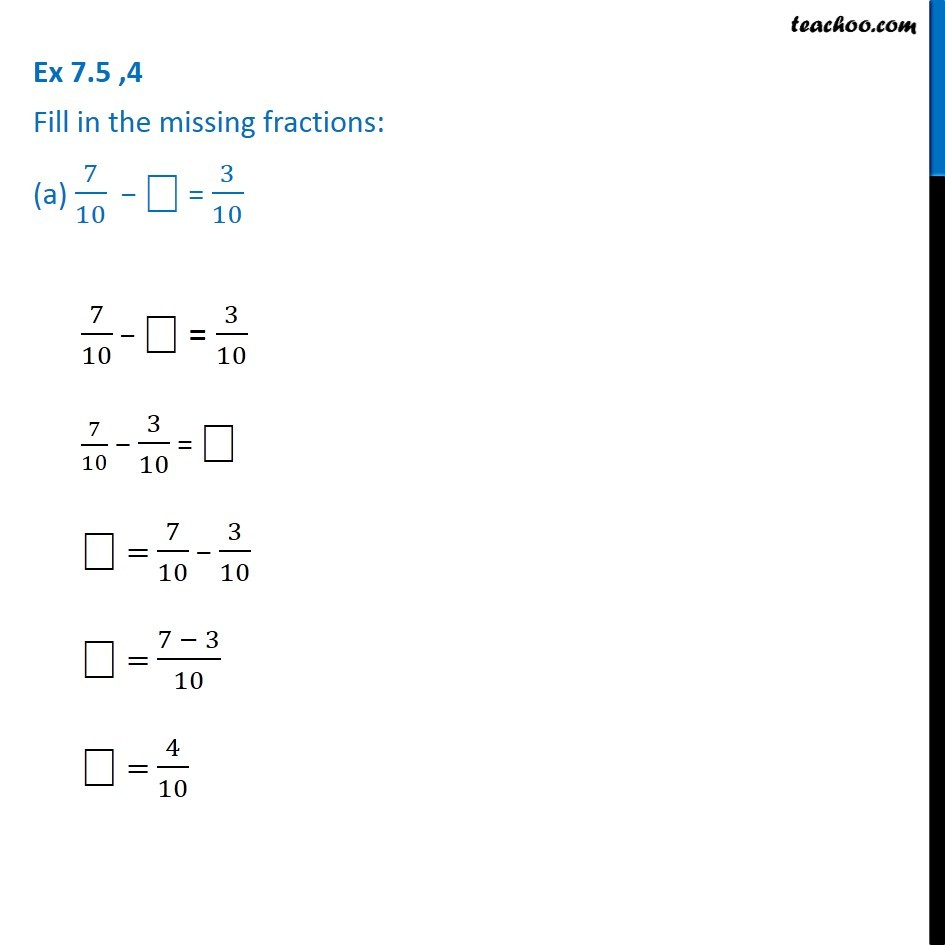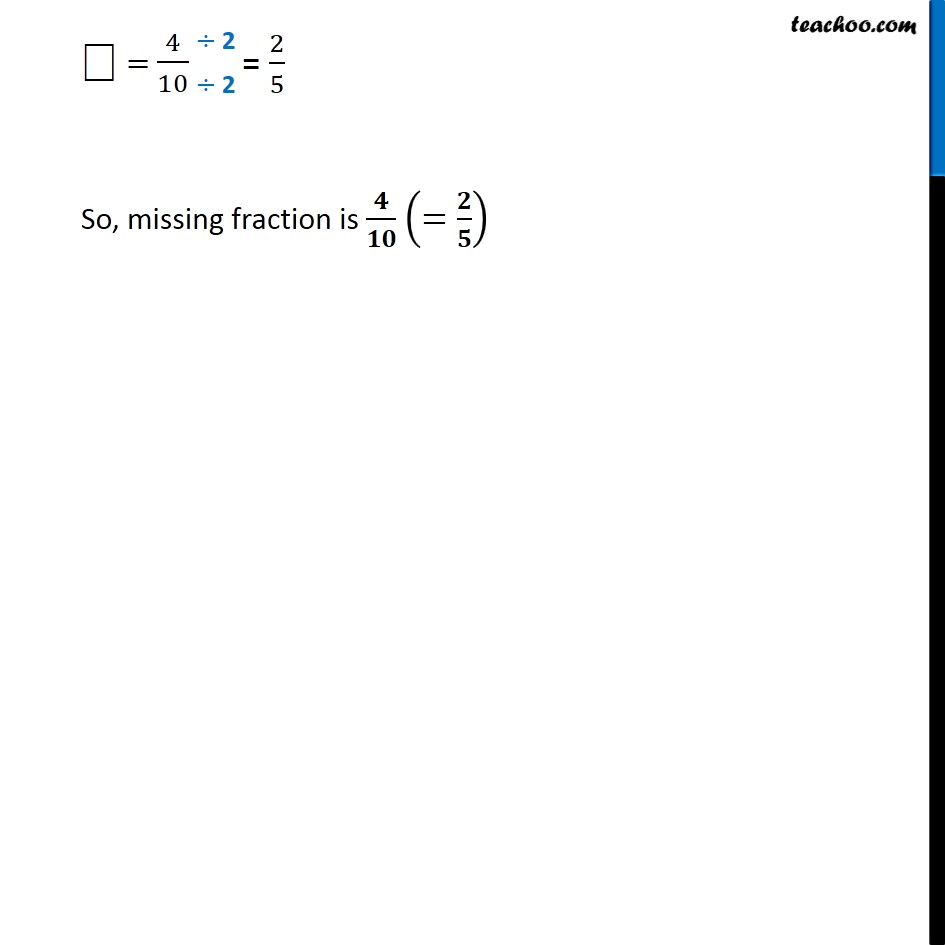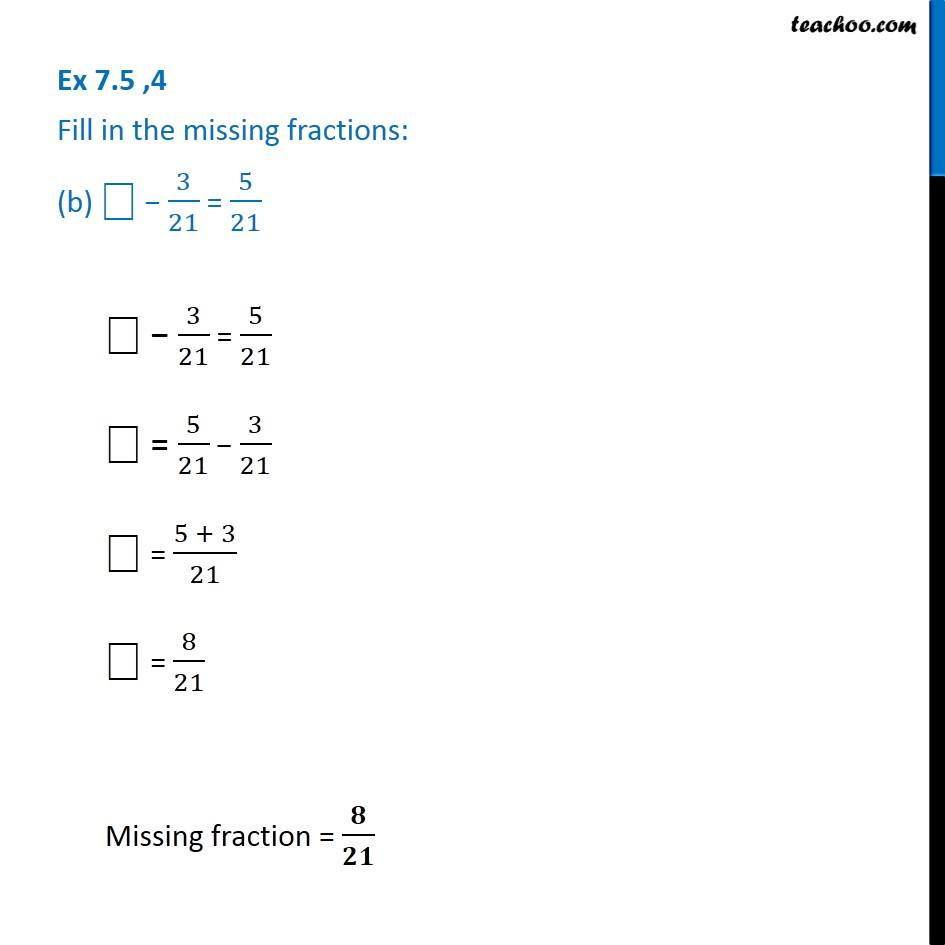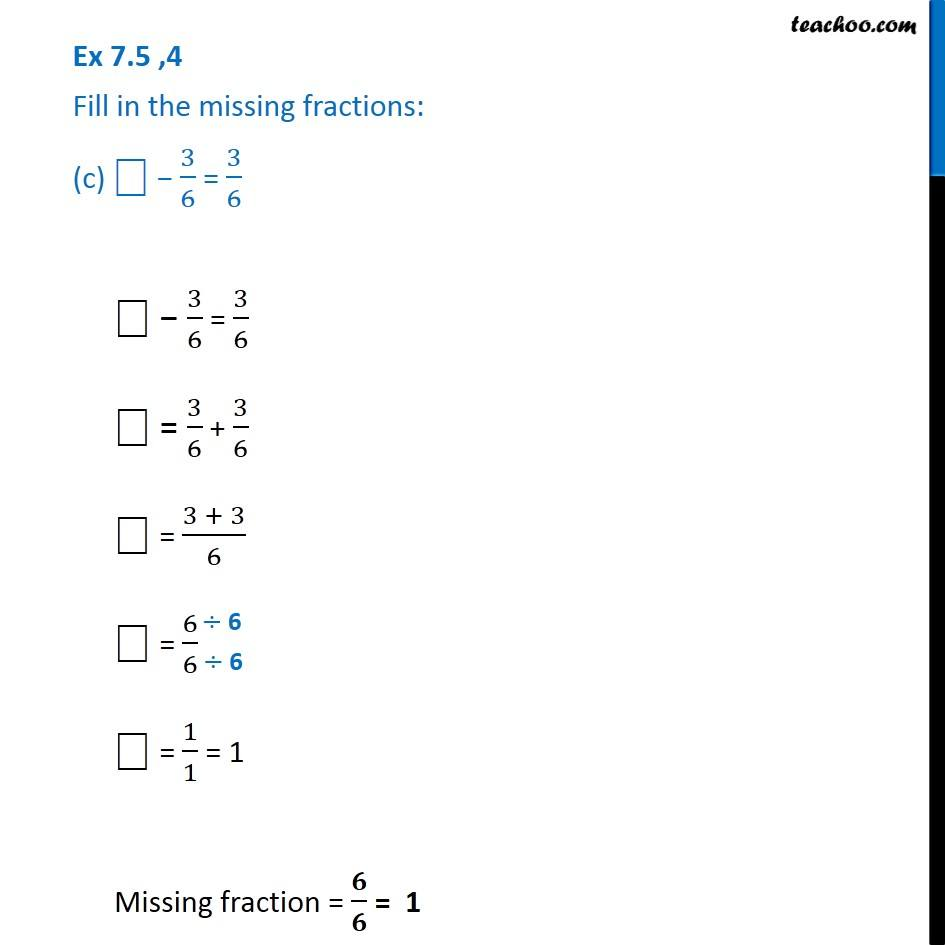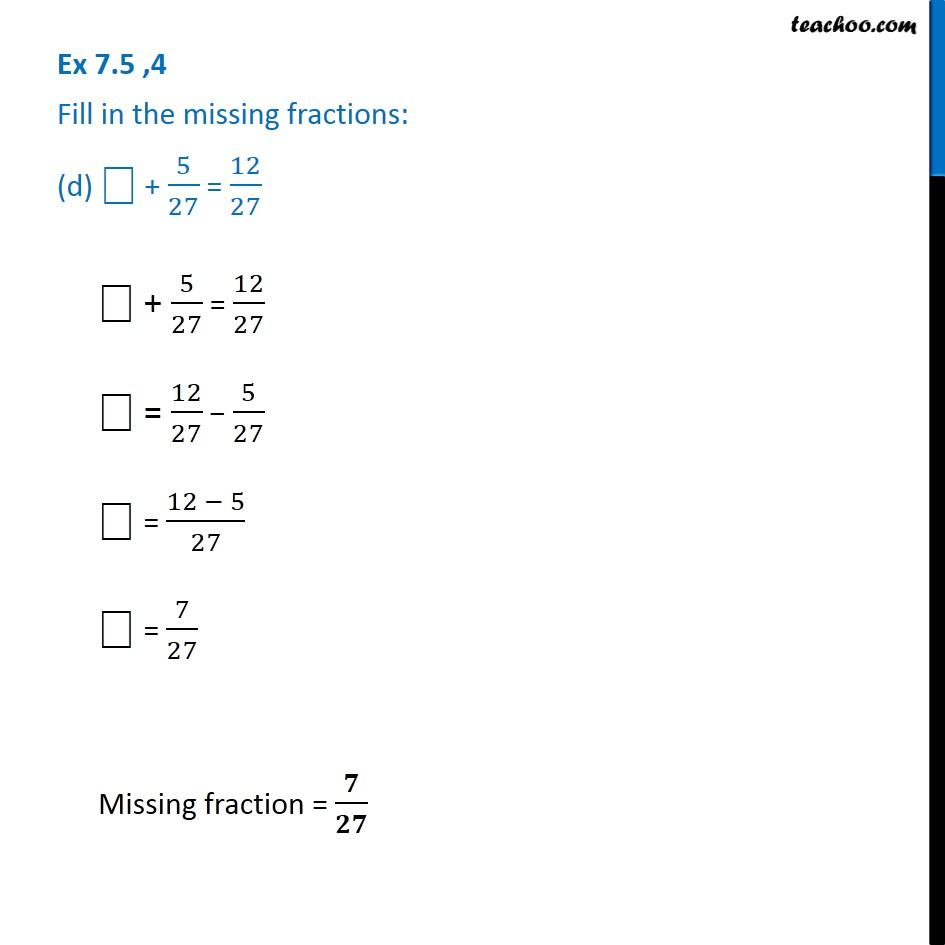Subscribe to our Youtube Channel - https://you.tube/teachoo

1. Chapter 7 Class 6 Fractions
2. Concept wise
3. Addition and Subtraction of Like Fractions

Transcript

Ex 7.5 ,4 Fill in the missing fractions: (a) 7/10 − ⎕ = 3/107/10 − ⎕ = 3/10 7/10 − 3/10 = ⎕ ⎕ = 7/10 − 3/10 ⎕ = (7 − 3)/10 ⎕ = 4/10 ⎕ = 4/10 So, missing fraction is 𝟒/𝟏𝟎 (=𝟐/𝟓) Ex 7.5 ,4 Fill in the missing fractions: (b) ⎕ − 3/21 = 5/21⎕ − 3/21 = 5/21 ⎕ = 5/21 − 3/21 ⎕ = (5 + 3)/21 ⎕ = 8/21 Missing fraction = 𝟖/𝟐𝟏 Ex 7.5 ,4 Fill in the missing fractions: (c) ⎕ − 3/6 = 3/6 ⎕ − 3/6 = 3/6 ⎕ = 3/6 + 3/6 ⎕ = (3 + 3)/6 ⎕ = 6/6 ⎕ = 1/1 = 1 Missing fraction = 𝟔/𝟔 = 1 Ex 7.5 ,4 Fill in the missing fractions: (d) ⎕ + 5/27 = 12/27 ⎕ + 5/27 = 12/27 ⎕ = 12/27 − 5/27 ⎕ = (12 − 5)/27 ⎕ = 7/27 Missing fraction = 𝟕/𝟐𝟕

Addition and Subtraction of Like Fractions

About the AuthorDavneet Singh
Davneet Singh is a graduate from Indian Institute of Technology, Kanpur. He has been teaching from the past 9 years. He provides courses for Maths and Science at Teachoo.